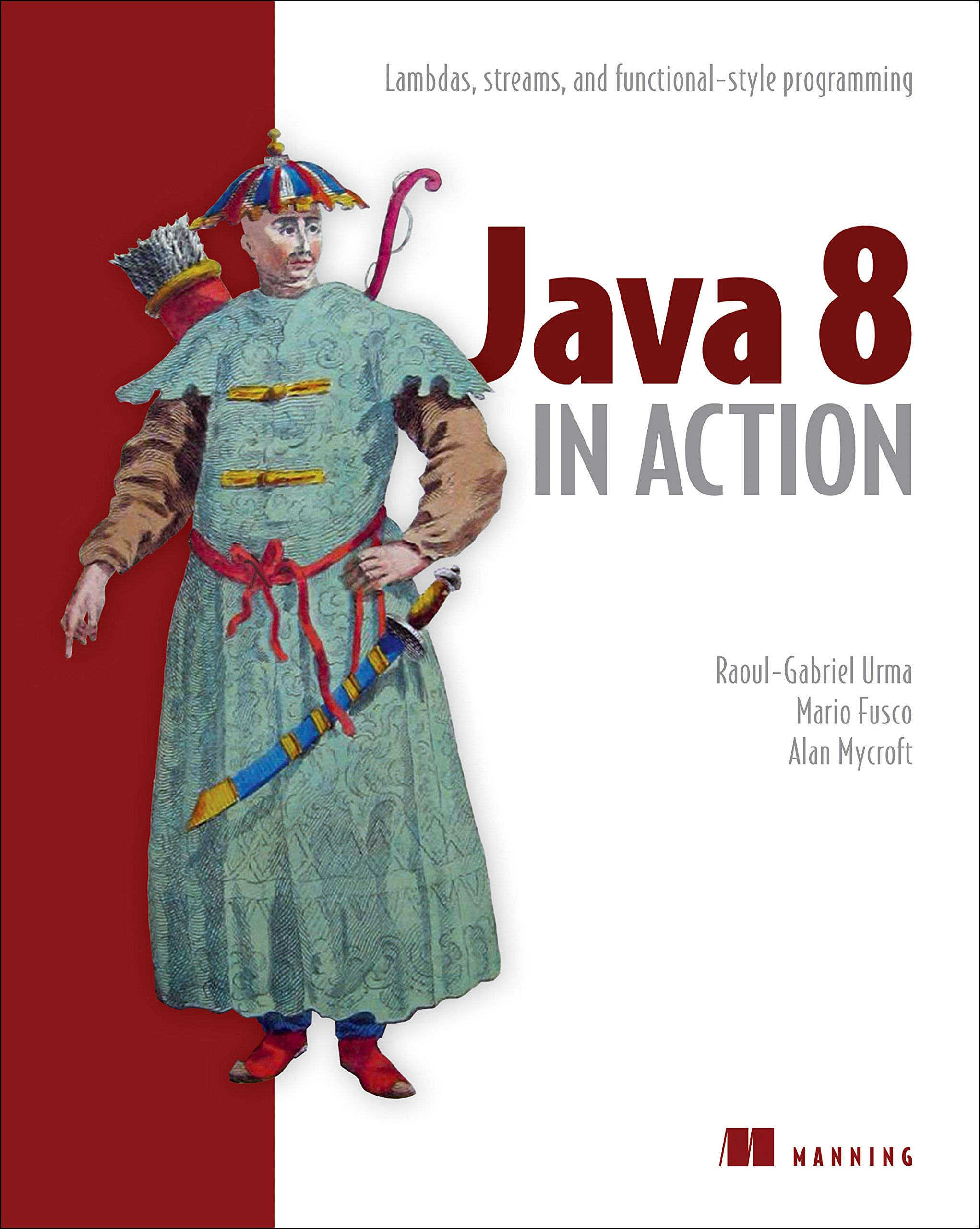Java 8 - Using Functions as Values - The Koch Family

جاري التحميل ...

# Java 8 - Using Functions as Values

The following is a series of posts about "functional programming in Java" which is the result of my understanding by reading the book "Java 8 in Action: Lambdas, Streams, and Functional-style Programming, by Alan Mycroft and Mario Fusco".
1. Why functional programming?
2. Functional programming in Java 8
3. Java 8 - Using Functions as Values
4. Java 8 - Persistent data structure

In general, the phrase "functional-style programming" means the behavior of functions should be like that of mathematical-style functions - no side effects.

In programmers' point of views, functions may be used like other values: passed as arguments, returned as result, and stored in data structures. That means we can use the :: operator to create a method reference, and lambda expressions to directly express function values. For example:
`//method referenceFunction<String, Integer> strToInt = Integer::parseInt;//lambda expressionComparator<Integer> comparator = (a, b) -> a.compareTo(b);`

There are two practical techniques to apply in our codebase.

## Higher-order functions

For example:
` Comparator<Apple> c = Comparator.comparing(Apple::getWeight);`
Higher-order functions (like Comparator.comparing) that can do at least one of following:
• Take one or more functions as parameter
• Return a function as result
Foo: If so, for what?
Bar: Good question! To compose functions to create a pipeline of operations. It's close to declarative programming.

## Currying

A function of two arguments (x and y, say) is seen instead as a function g of one argument that returns a function also one argument. The value returned by the latter function is the same as the value of the original function, that is, f(x,y) = (g(x))(y).

Brain overload! For example, you want to convert Celsius to Fahrenheit, the formula is f(x) = x *9/5 + 32. Not only this case but we can see the basic pattern of all unit conversion is as follow:
`static double converter(double x, double f, double b) { return x * f + b;}`
This method is a bit too general. Obviously, we can use it to apply for all unit conversion but it would be tedious and you might accidentally mistyped them. There is an better approach:
`static DoubleUnaryOperator curriedConverter(double f, double b){ return (double x) -> x * f + b;}`
Instead of passing all the arguments x, f and b all at once to the converter method, you only ask for the arguments f and b and return another function, which given an argument x return x*f + b.

Why is it the better approach? This enables you to reuse the convention logic and create different functions with different convention factors:
`DoubleUnaryOperator convertCtoF = curriedConverter(9.0/5, 32);DoubleUnaryOperator convertUSDtoGBP = curriedConverter(0.6, 0);DoubleUnaryOperator convertKmtoMi = curriedConverter(0.6214, 0);`
Instead of three different method for each conversion factor:
`static double convertCtoF(double x, double f, double b) { return x * f + b;}static double convertUSDtoGBP(double x, double f, double b) { return x * f + b;}static double convertUSDtoGBP(double x, double f, double b) { return x * f + b;}`
Foo: If so, for what?
Bar: It helps you modularize functions and reuse code!

Reference. Alan Mycroft and Mario Fusco, Java 8 in Action: Lambdas, Streams, and Functional-style Programming. Part 4. Beyond Java 8.

If you like the content of our blog, we hope to stay in constant communication, just enter your email to subscribe to the blog's express mail to receive new blog updates, and you can send a message by clicking on the button next ...

## إتصل بناThe Koch Family <<  Welcome! I'm so glad that you stopped by Your Modern Family blog. Together, we will talk about raising kids, organizing the home and saving money! and Tips & tricks and more…2513183